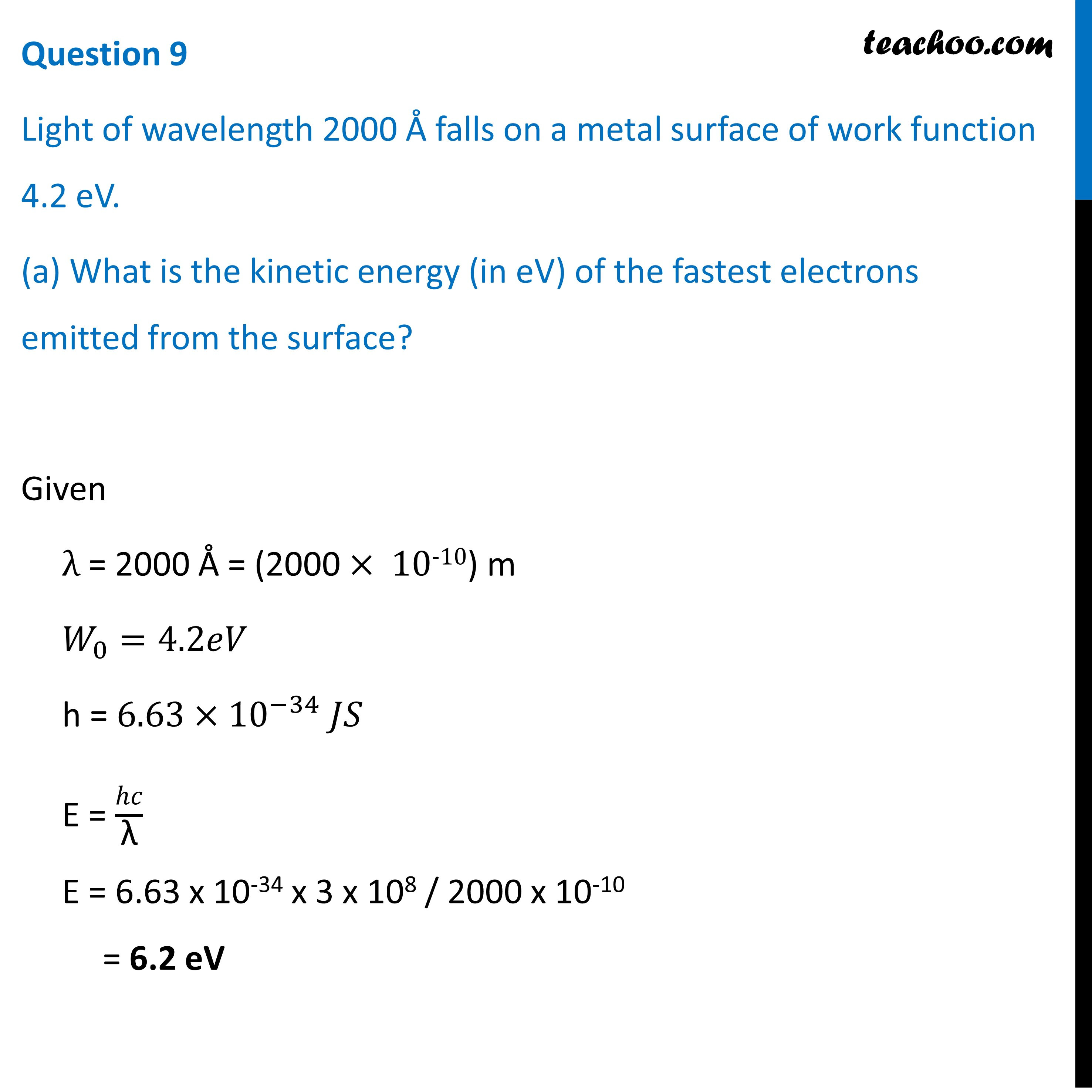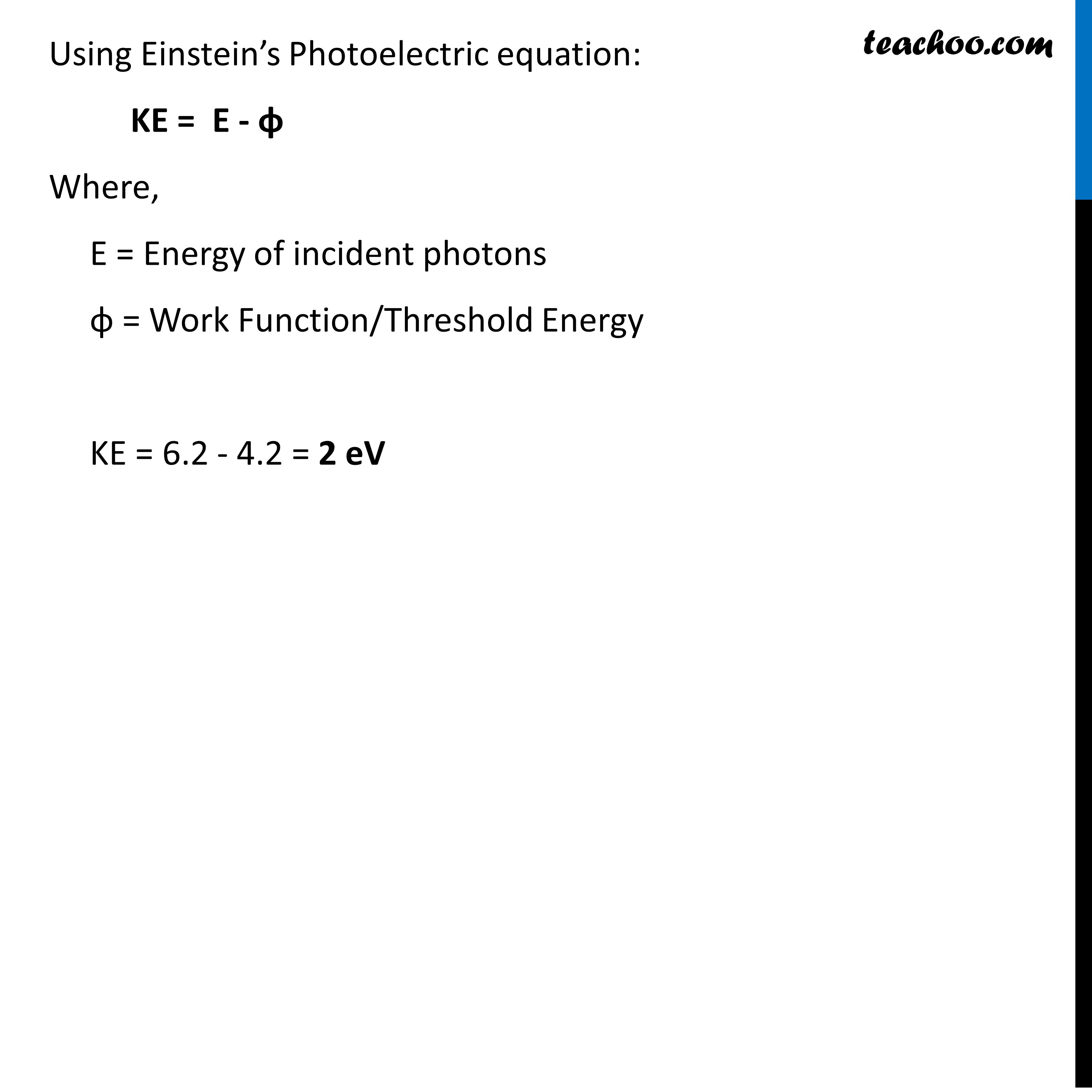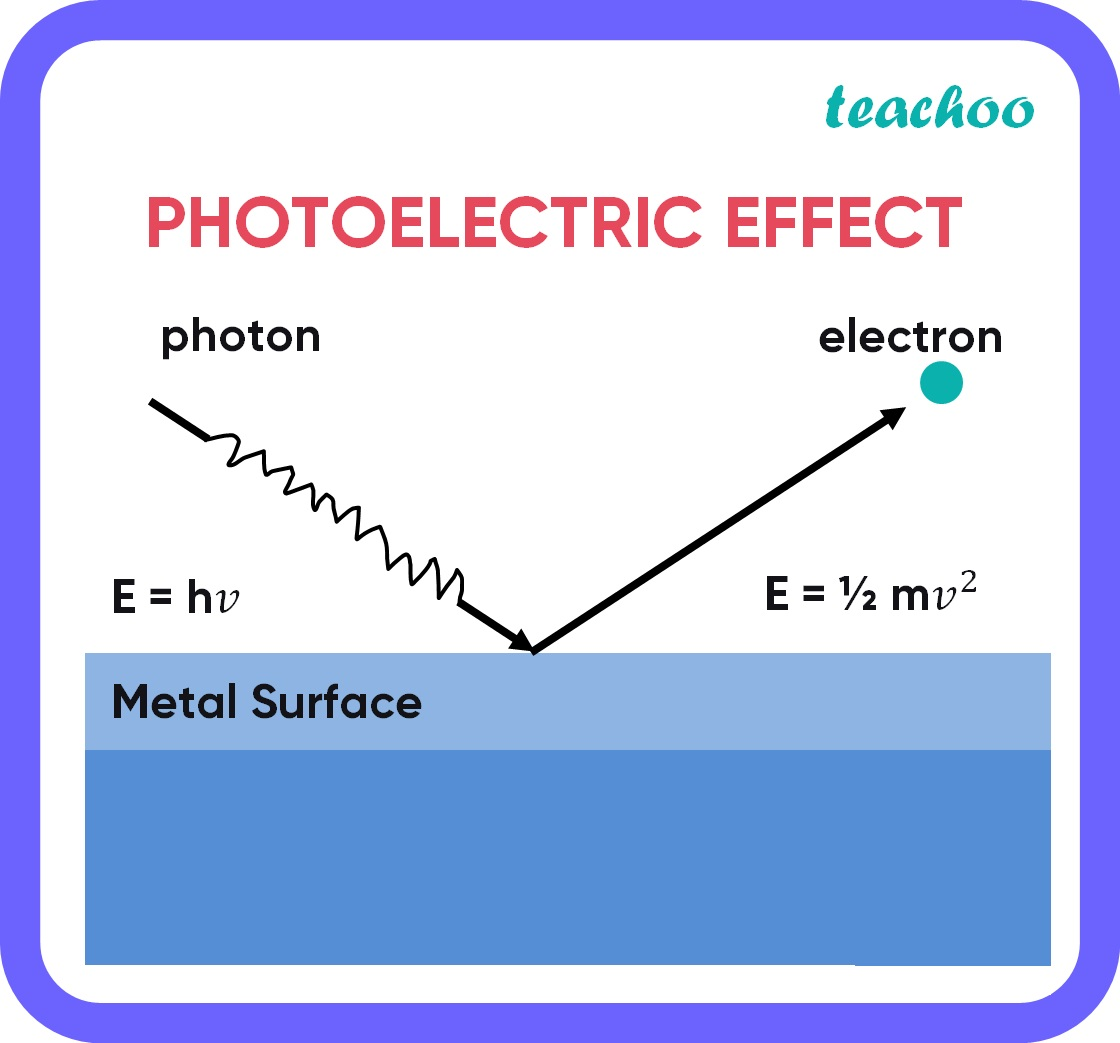CBSE Class 12 Sample Paper for 2022 Boards [Term 2] - Physics

Physics Class 12
Solutions to CBSE Sample Paper - Physics Class 12

## (c) If the same light falls on another surface of work function 6.5 eV, what will be the energy of emitted electrons?

(a)(b) Intensity of light is defined as the power of light per unit solid angle or the energy of light per unit time, per unit area.

• The energy of emitted electrons is independent of the intensity of light.
• Hence, the energy of emitted electrons does not change

(c) Threshold energy is the minimum energy required to remove electrons from the surface of a metal. It is also known as the work function.

Here, work function of the surface is 6.5 eV

Since the energy of incident photons is less than the work function of the surface, no photoelectrons will be emittedLearn in your speed, with individual attention - Teachoo Maths 1-on-1 Class

### Transcript

Question 9 Light of wavelength 2000 Å falls on a metal surface of work function 4.2 eV. (a) What is the kinetic energy (in eV) of the fastest electrons emitted from the surface? Given λ = 2000 Å = (2000 × 10-10) m 𝑊_0=4.2𝑒𝑉 h = 6.63 × 10^(−34) 𝐽𝑆 E = ℎ𝑐/"λ" E = 6.63 x 10-34 x 3 x 108 / 2000 x 10-10 = 6.2 eV Using Einstein’s Photoelectric equation: KE = E - ɸ Where, E = Energy of incident photons ɸ = Work Function/Threshold Energy KE = 6.2 - 4.2 = 2 eV (b) What will be the change in the energy of the emitted electrons if the intensity of light with the same wavelength is doubled? (c) If the same light falls on another surface of work function 6.5 eV, what will be the energy of emitted electrons? b) Intensity of light is defined as the power of light per unit solid angle or the energy of light per unit time, per unit area. The energy of emitted electrons is independent of the intensity of light. Hence, the energy of emitted electrons does not change. (c) Threshold energy is the minimum energy required to remove electrons from the surface of a metal. It is also known as the work function. Here, work function of the surface is 6.5 eV Since the energy of incident photons is less than the work function of the surface, no photoelectrons will be emitted.